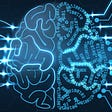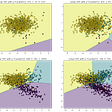# Recap

In the previous posts we showed how connecting several perceptrons together can be a powerful way to find non linear decision boundaries.

But how do we ensure that the decision boundary we find will work well on unseen data?

# Overfitting and underfitting

To elaborate on the question posed above, consider the following models. Which would we consider most likely to succeed on classifying future data points (those that are not currently in our dataset) ?

We know that the one of the left is too simple, and such a model would be said to ‘under fit’ the data. In the context of MLPs this would mean our architecture isn’t complex enough ( we don’t have enough perceptrons and/or layers in our hidden layers to create a sophisticated enough model ).

The model on the right looks like it would do poorly if we introduced any new data. Remember these are supposed to be PREDICTIVE models. The whole point of this exercise is to use our labelled data points to find out a model which will take an unlabelled data point and classify it correctly. So while the model on the right would have the lowest average loss, it is not the best model.

A model which over fits to the data is like a student who has just memorised all the answers to a set of past papers, but has learnt none of the underlying concepts. They will always get 100% on the past papers but do poorly in an unseen test.

The model in the middle seems to be the ‘goldilocks’ model, where we’ve found a good balance of complexity and generalisability. This is the goal, we want to make sure after training, our model is sufficiently complex, but will also handle unseen data points well. We will now detail steps we can take to increase the likelihood of this being the case.

# Train/validate/test - splitting our dataset

So think back to the last post, the part where we discussed how these neural networks were trained.

Here’s a quick summary:

1. We take each data point in our data set, and run it through the model. This is the forward pass.
2. We calculate a loss via a loss function and then use gradient descent or another optimiser. This tells us the way in which we should update the weights and biases of our model to make it give a more accurate output for that data point.
3. We do this for all the data points in our data set, this is one epoch. We then update the weights and biases by averaging all the proposed changes. This reduces our average loss across our entire data set.

If we keep repeating the above over several epochs, we should end up with a lower and lower loss. But we need some way to check that the model isn’t over fitting to our data set at the same time. This is why, in practice, we split our dataset into 3 subsets:

Train set - this is the subset of the total data that the network will see during training (the above loop) , typically 70% of the total data.

Validation set - this is the subset of the total data that will be used to test the models performance during training, typically 10% of the total data.

Test set - this is the subset of the total data that will be used to test the final models performance, typically 20% of the total data.

Let’s break each set down using our previous analogy of a student studying for a test.

The train set is the problem sheet set that the student is assigned to learn the concepts and become better at solving the type of problems that could show up on the final. For our model, the train set is the data it uses to actually update its weights and biases to reduce the loss. It learns from this dataset what values to assign to its parameters such that it can keep the loss low.

The validation dataset would be past papers that the student can use to test their knowledge of the underlying concepts. If the student just memorised the answers to the problem sheet, they’ll do poorly. If they actually learnt the concepts then they will do well. For our model, we test its performance on the validation dataset after every epoch ( after every complete pass of the training dataset through the model ). We do not update the weights and biases of our model based on the validation loss. We simply use this as a way to gauge its performance on unseen data. We expect to see that as the training continues the validation loss will decrease. If we see the validation loss increasing, we know we are now overfitting to the training data.

The final data set is the train set, this is the final exam the student must take to get a grade for the course. For our model, this is the final data set we use to test its real world performance. This dataset can only be used once. If it turns out the models performance is inadequate we must go back through this entire process of training, validating and testing a new model.

## Validation vs testing

OK, so we can flag for over fitting by holding back part of our data and validating our model during training. So why do we need the 3rd set, the testing set?

The test set is used as the final data set to test our final trained models accuracy. Well, whats stopping us from just using the validation data set to test our final model as well? After all, the model hasn’t used this data set to update its weights and biases, so as far as the model is concerned, it’s unseen data.

In practice, what is done is we actually train a bunch of different models. These models could have different architectures, or they could just have a different set of hyper parameters ( more on this in the next article ). The point being, we normally have a bunch of candidate models we train, we pick the model that has the lowest validation loss as our final model. We still need to be sure that our change lead to a genuinely better model, as compared to a model which just fits well to the validation set.

Let’s break it down further. Imagine you are training a model to classify some digits from the MNIST data set. You’ve broken the data down into training and validation. You’ve picked your model’s architecture and a set of hyper parameters. Now you train the model and after every epoch, test it on the test set. You will see the validation loss decrease as your training continues. Great! At some point your validation loss will either plateau or start go back up. At this point you want to stop your training. Now you go and think, maybe if just add another hidden layer or increase the learning rate, i could get a lower final loss. So you go back, make some tweaks to the model and re train and test. You keep doing this till eventually your final validation loss is pretty low and you’re happy with the result.

Now the problem with the above situation is that, by having gone in at the end, looking at the validation loss and then making changes to our model, we’ve introduced human bias. We’ve manually made changes to our model to optimise on a specific data set. We still have yet to see whether the gains made on the validation loss are due to a superior model or due to our model being optimised on that data set. This is what the test set is for. It is for an unbiased evaluation of our model. If it fails to perform adequately on our test set, we simply re do the training, validation and test process same as before. But now we test on a new dataset. This way we can be sure our models performance is genuinely improving and its not just becoming more optimised on a specific set of data.

# Summary

In this article we introduced the reasoning behind the train, validate and test split approach to avoid over fitting. This is good way to ensure the trained model generalises to unseen data. This is the first article in the series on training neural networks. The next will cover how to handle class imbalances and the final article will cover different types of hyper parameters and how they affect training.

## More from Analytics Vidhya

Analytics Vidhya is a community of Analytics and Data Science professionals. We are building the next-gen data science ecosystem https://www.analyticsvidhya.com

## End to End Recipe Cuisine Classification## Reinforcement Learning Explained Visually (Part 3): Model-free solutions, step-by-step## Sentiment Analysis and Emotion Recognition in Italian (using BERT)## My Journey Into Machine Learning as a High School Student## Building a Deep Learning Person Classifier## Sentiment Analysis:## H2O.ai Launches Python Framework to Develop Artificial Intelligence Apps## A Machine Learning Approach to Predict Diabetic Patient Hospital Readmissions (contd..) — Part 15## Vishal Jain

Final year physics @ Bath

## A common mistake to avoid in Machine Learning projects## Data sampling methods for imbalanced data## About Ensemble Techniques in ML.## What does this additional term in the linear regression equation mean?